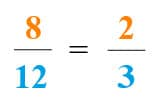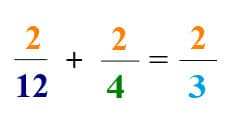The K5 Learning Blog urges parents to be pro-active in helping their children reach their full academic potential.

K5 Learning
provides free worksheets, workbooks and an online reading and math program for kindergarten to grade 5 students.# How to add fractions with unlike denominators

Following on from our last blog on adding fractions with like denominators, adding fractions with unlike denominators is a little more complicated.

Improper fractions, or fractions with unlike denominators, may look a bit difficult. However, once you make the denominators the same, the addition is easy.

Let’s use an example: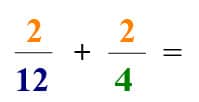## Find the least common multiple

First, we need to find the Least Common Multiple (LCM) for the denominators. Basically, we need to find a common multiple that they share.

The common multiples of 4 are 4, 8, 12, etc.

The common multiples of 12 are 12, 24, 36, etc.

Now we need to find the first common value. For most students new to this, it’s easiest to sketch it out on a number line: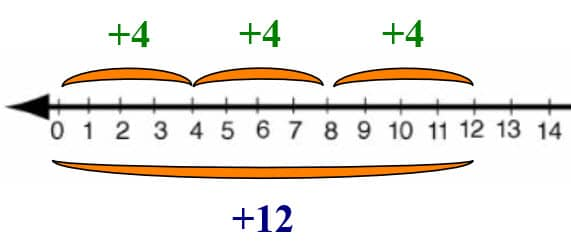The least common multiple is (1, 3) = 12.

## Multiply the numerator and denominator to get like denominators

Now, you’ll need to multiply the entire fraction to make the denominator become the least common multiple. In our example, this is what that looks like: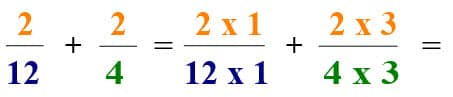Important: you multiply both the top and the bottom by the same amount to keep the value of the fraction the same.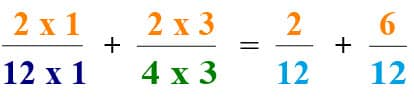Now the denominators (the bottom numbers) are the same. This means we can add the numerators (the top numbers):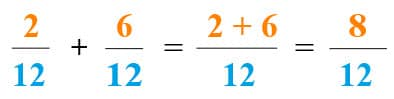## Simplifying fractions

Finally, let’s simplify the fraction.

Think about the Greatest Common Factor: the highest number that divides evenly into both the numerator and the denominator.

8 divides into 1, 2, 4, 8

12 divides into 1, 2, 4, 6, 12

The Greatest Common Factor is 4. 8/4 = 2, and 12/4 = 3: